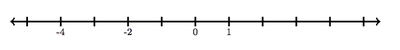# Integers on the Number Line 2

Alignments to Content Standards: 6.NS.C.6.a

Below is a number line with 0 and 1 labeled:1. Find and label the numbers $-2$ and $-4$ on the number line. Explain.
2. Find and label the numbers $-(-2)$ and $-(-4)$ on the number line. Explain
3. Find and label the number $-0$ on the number line. Explain.

## IM Commentary

The goal of this task is to study, with a number line, why it makes sense for a whole number $a$ that  $-(-a) = a$. The negative sign preserves the distance from 0 but switches the direction from 0. There are only two directions to go on the number line: left and right.  Therefore, if we switch directions twice this will bring us back to our original direction from 0.  Since the distance from 0 does not change, this means that taking the opposite of a negative number will produce a positive number with equal distance from 0.  This reasoning will be foundational for future 7th grade work where students must reason about the opposite of quantities such as (-2 + 8) and -(-2 + 8).

This task complements https://www.illustrativemathematics.org/illustrations/283 which investigates plotting negative integers on the number line.

## Solution

1. The numbers -2 and -4 are both negative and so they are both located to the left of 0. To place -2 on the number line, we need to move two units to the left of 0 and for -4 we move four units to the left of 0. The unit has been marked on the number line (in the positive direction) so we can count 2 and 4 equal tick marks to place -2 and -4 as shown below:2. For -(-2) we are looking for the negative or opposite of -2. Since -2 is two units to the left of 0 this means that -(-2) will be two units to the right of 0. Similarly -(-4) is 4 units to the right of 0. These are plotted on the number line below:Notice that -(-2) = 2 and -(-4) = 4. The first negative sign switches us from the right of 0 to the left of 0 and the second negative sign puts us back where we started.

3. Since 0 is neither to the right nor to the left of 0, taking the opposite of 0 will give 0, that is -0=0. For a positive number, such as 2, the opposite or -2 is a negative number. For a negative number such as -4, the opposite or -(-4) is positive. The number 0 is neither positive nor negative so we must have -0=0.